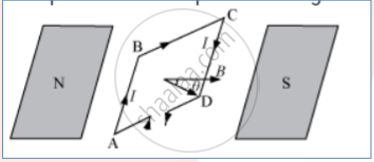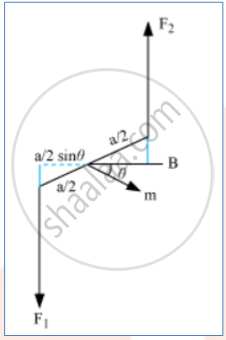# Derive the Expression for the Torque Acting on a Current-carrying Loop Placed in a Magnetic Field. - Physics

#### Question

Derive the expression for the torque acting on a current-carrying loop placed in a magnetic field.

#### Solution

The plane of the loop is not along the magnetic field but makes an angle with it.Let the dimension of the rectangular coil ABCD, be AB × BC = a × b
The angle between the field and the normal is θ.
Forces on BC and DA are equal and opposite and they cancel each other as they are collinear.
Force on AB is F1 and force on CD is F2.

F1 = F2 = IbB

The magnitude of the torque on the loop as in the figure:tau = F_1 a/2 sinθ + F_2 a/2 sinθ

= IabBsinθ

If there are ‘n’ such turns the torque will be sin nIab sinθ

The magnetic moment of the current m = IA

vectau = vecm xx vecB

Concept: Torque on a Current Loop in Magnetic Field
Is there an error in this question or solution?